×

×

ISU / Chemistry / CHE 140 / It is the flow of solvent through a semi-permeable membrane to equaliz

# It is the flow of solvent through a semi-permeable membrane to equaliz Description

##### Description: Study Guide covers the material covered from Chapter 9 through Chapter 11
10 Pages 56 Views 13 Unlocks
Reviews

German Guzman (Rating: )

svander824@gmail.com (Rating: )

Kristy Mahal (Rating: )

CHE140 Exam 4 Study Guide

## It is the flow of solvent through a semi-permeable membrane to equalize the concentration of two solutions, what is it?Chapter 9 (9.7)

Sigma σ versus Pi π bonds

∙ Sigma σ bonds

o The orbitals overlap between the nuclei S+S

o Sigma bonds occur in the bonding molecular orbitals

∙ Pi π bonds

o The orbital overlap does not occur between the nuclei, but above and below o Pi bonds occur in the anti-bonding molecular orbitals If you want to learn more check out What do you call a sheath that fits over the penis and is used to block sperm from reaching an ovum?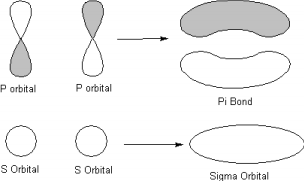## What is the difference between diamagnetic and paramagnetic?Definitions/Concepts

∙ Diamagnetic – a molecule that has only paired electrons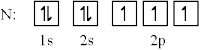∙ Paramagnetic – a molecule that has unpaired electrons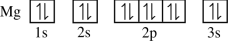## What is ion-dipole interactions?If you want to learn more check out In what year the indian savage idea was challenged with little big man?

MO Theory

∙ Overlap of Orbitals If you want to learn more check out What do you call to the sense of smell, which occurs when receptors in the nose respond to chemicals?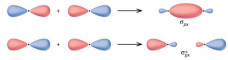We also discuss several other topics like How is anthropology a field of mediation?

o The top diagram is an example of a constructive overlap (bonding orbital – hold  the molecule together)

o The bottom is an example of a destructive overlap (anti-bonding orbital – discourage the formation of bonds/break them apart [in the molecular orbital  diagram, notated with an asterisk *])

∙ General Shapes of Overlaps

o S-S sigma bonding and anti-bonding We also discuss several other topics like What type of operations usually get the best pricing?o P-P sigma bonding and anti-bonding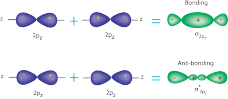o P-P pi bonding and anti-bonding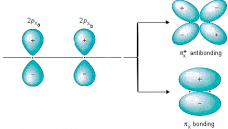If you want to learn more check out Enzymes comes from the greek word what?

∙ Molecular Orbital Diagrams

o Fill in molecular orbital diagrams with valence electrons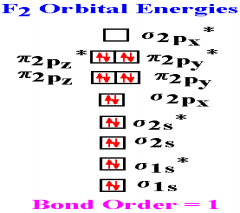∙ Calculating Bond Order

o Bond order is calculated by:

[Number of electrons in bonding MOs] – [Number of electrons in anti-bonding MOs]  2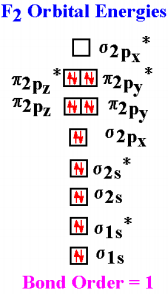▪ In this example, there are 10 electrons in bonding MOs and 8 electrons in  the anti-bonding MO’s (remember the *). Subtract 8 from 10 to get 2, then  divide by 2. The bond order is 1.

∙ Correlating bond order, bond length, and bond strength

o As the bond order of a compound increases the bond strength increases and the  bond length decreases

Bond

# of electrons

Bond Order

Bond Strength

Bond Length

Single

2

1

Weakest

Longest

Double

4

2

Triple

6

3

Strongest

Shortest

Chapter 10

Definitions/Concepts

∙ Hydrophilic – water-loving (dipole-dipole and hydrogen bonding)

∙ Hydrophobic – water-fearing (London dispersion)

∙ Supercritical fluid – any substance at a temperature and pressure above its critical point,  where distinct liquid and gas phases do not exist

∙ Meniscus – the curve in the surface of liquid close to the surface of the container or  another object, caused by surface tension

∙ Adhesive force – an upward meniscus caused by unalike forces (mercury in a graduated  cylinder

∙ Cohesive force – a downward meniscus caused by like forces (H2O in a graduated  cylinder)

Ion-Ion Forces

∙ Hold ions together in ionic solids

o Found by: Eel = Q1Q2

d

∙ The larger the charge on the ions, the stronger the ion-ion forces

o Larger charges also cause the ions to be less soluble

∙ The larger the size of the ion, the weaker the ion-ion forces

Ion-Dipole Interactions

∙ An Ion-Dipole interaction is the electrostatic attraction of an ion with the dipole of a  polar molecule

o Not as strong as an ion-ion force

∙ Cations (+) are attracted to the partially negative ends of polar solvent molecules such as  water (Na+ would be attracted to the O of a water molecule)

∙ Anions (-) are attracted to the partially positive ends of polar solvent molecules such as  water (Cl- would be attracted to the H of a water molecule)

∙ Sphere of Hydration – each ion interacts with multiple (generally 4-6) solvent molecules

Dispersion Forces (London Dispersion Forces)

∙ The only intermolecular force present in non-polar molecules; present in ALL molecules ∙ Weakest intermolecular force

∙ Caused by temporary dipoles

o A temporary dipole is caused by a temporary upset due to an uneven distribution  of electrons (happens very fast)

∙ Polarizability – the tendency of the electrons in an atom or molecule to be distorted,  creating a temporary dipole

o The more electrons in a molecule, the more polarizable it is and the stronger the  dispersion force

Dipole-Dipole Interactions (think of magnets)

∙ Present in polar molecules

∙ This is the electrostatic attraction between oppositely charged ends (dipoles) in molecules ∙ The larger the dipole (the more polar the molecule), the stronger the dipole-dipole  intermolecular force

Hydrogen Bonds

∙ The strongest of the intermolecular forces

∙ A very strong dipole-dipole force

∙ Hydrogen bonding is present when hydrogen bonds directly to oxygen, nitrogen, or  fluorine

Predicting Boiling Points/Melting Points

∙ Boiling points increase/freezing points decrease as the number of carbons is increased ∙ Boiling points decrease/freezing points increase as molecules branch

“Like Dissolves Like”

∙ Polar compounds are soluble in polar solvents

o Sugar is a polar compound, and therefore soluble in polar solvents such as water ∙ Non-polar compounds are soluble in non-polar solvents

o Naphthalene is a non-polar compound, and therefore soluble in non-polar solvents  such as hexane

Temperature/Pressure and Gases

∙ All gases are less soluble as the temperature increases

o The solubility of gases increases with increasing pressure

Henry’s Law

∙ Cgas = KH x Pgas

o K is the constant

o P is the pressure of the gas

Phase Diagram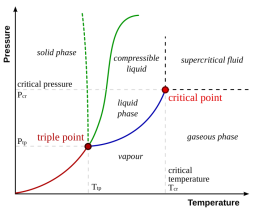∙ The red line is the line that represents going from solid to gas (sublimation) ∙ The blue line is the line that represents going from liquid to gas (boiling) ∙ The green line is the line that represents going from solid to liquid (melting) ∙ Triple point – all three phases are present at the same time

∙ Critical point – the end point of the phase diagram (anything past this is called the  supercritical region, which contains supercritical fluid)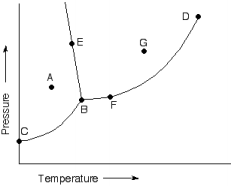What do the letters represent?

a. Solid

b. Triple point

c. Sublimation

d. Critical point

e. Melting

f. Boiling

g. Liquid

Properties of Water

∙ Surface tension – a liquid’s resistance to increasing its surface area

∙ Viscosity – a liquid’s resistance to flow

∙ The stronger the intermolecular forces, the higher the viscosity and the greater the surface  tension

∙ Surface tension is responsible when an object or insect that is denser than water is able to  float or run along the water surface

Chapter 11

Enthalpy of Solution and Hydration

∆Hsol = ∆Hion-ion + ∆Hsolvent-solvent +∆Hion-dipole

∙ ∆Hion-ion (energy in) is the lattice energy (u)

o u = K x Q1 x Q2

d

∙ ∆Hsolvent-solvent + ∆Hion-dipole (energy out) is ∆Hhydration (hydration enthalpy) ∆Hsol = ∆Hhyd – u

∙ Calculate u using the Born-Haber Cycle

Na(g) + ½ Cl (g) ???? NaCl(s) ∆Hof

Na+(aq) + Cl-(aq) ???? NaCl(s) ∆H = u

∙ Whether using the standard enthalpy of formation or the lattice energy, you get NaCl

Lattice Energy, u

∙ (K x Q1 x Q2) / d

∙ Calculate using the Born-Haber Cycle

Born-Haber Cycle for 2Na(s) + ½ O2(g) = Na2O(s)

2Na+(g) + O2-(g)

(3). 2(IE) (5). EA1 + EA2 u

2Na+(g) + O(g)

(2). 2(∆Hsub) (4). ½ BDE

2Na(s) + ½ O2(g)

(1.) ∆Hof

Na2O(s)

∆Hof = 2(∆sub) + 2(IE) + ½ (BDE) + (EA1 + EA2) + u

-416 = 2(107) + 2(496) + ½ (499) + 603 + u

-416 = 2058.5 + u

u = -2475 kJ/mol

Vapor Pressure

∙ Vapor pressure is the amount of a substance in the gas phase above a liquid ∙ The stronger the intermolecular forces, the lower the vapor pressure in pure liquids

o SOLUTIONS will have a lower vapor pressure than the pure solvent

Molality (cursive lowercase m)

∙ Moles of solute

kg solvent

Colligative Properties

∙ Van’t Hoff Factor, i

o A measure of the number of particles in solution per molecule/formula unit o MgCl2 dissolves into one Mg2+ cation and two Cl anions

▪ The ideal van’t Hoff Factor is 3

∙ Boiling Point Elevation

o ∆Tb = i x Kb x molality

∙ Freezing Point Depression

o ∆Tf = i x Kf x molality

Osmosis and Osmotic Pressure (π)

∙ Osmosis – the flow of solvent through a semi-permeable membrane to equalize the  concentration of two solutions

∙ Osmotic Pressure – the amount of pressure needed to stop osmotic flow o π = i x M x R x T

▪ i is the van’t Hoff Factor

▪ M is the molarity

▪ R is the ideal gas constant (0.0821 L x atm/ mol x K)

▪ T is the temperature in Kelvin

o ∆π = D(i x M) x R x T

▪ D(i x M) is the difference of the two concentrations

▪ i1 x M1 – i2 x M2

∙ Reverse Osmosis – applying a pressure greater than π to reverse the flow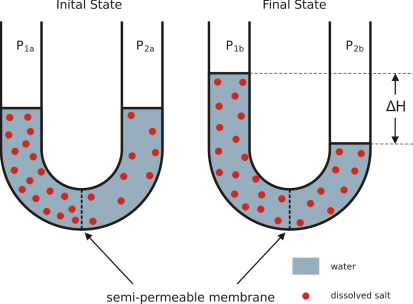Calculating Molar Mass Using Colligative Properties

∙ A solution of 19.4mg of a PCB in 1.00g CCl4 has its freezing point lowered by 1.60oC.  What is the molar mass of the PCB?

Kf = 29.8oC/molality

∆Tf = Kf x molality

Molality = ∆Tf/Kf = 1.60oC/29.6oC/molality = 0.053691molality

Molality = moles PCB

Kg CCl4

Moles PCB = molality x kg CCl4

= (0.053691)(0.00100kg)

Moles PCB = 5.3691 x 10-5 mol PCB

MM = g/mol = 0.0194g PCB

5.3691 x 10-5 mol PCB = 361 g/mol

Fractional Distillation

∙ Mixture of liquids can be distilled (boiled off)

∙ The composition of the vapor above a mixture of liquids has a different composition o Each solvent has a different vapor pressure

∙ The composition of the vapor above a mixture of liquids is determined by Raoult’s law o Psolution = Xsolvent x Posolvent

o Xsolvent is the mole fraction

o Posolvent is the vapor pressure

Page ExpiredIt looks like your free minutes have expired! Lucky for you we have all the content you need, just sign up here